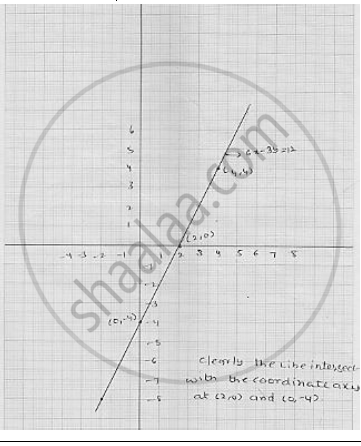# Draw the Graph of the Equation Given Below. Also, Find the Coordinates of the Point Where the Graph Cuts the Coordinate Axes : 6x − 3y = 12 - Mathematics

Draw the graph of the equation given below. Also, find the coordinates of the point
where the graph cuts the coordinate axes :  6x − 3y = 12

#### Solution

we have

6x − 3y = 12

⇒       3 (2x - y ) = 12

⇒        2x - y = 4

⇒        2x - 4 = y

⇒        y = 2x - 4         ................ (1)

Putting  x = 0 in (1) , we get  y =- 4

Putting  x = 2  in (1) , we get  y = 0

Thus, we obtain the following table giving coordinates of two points on the line
represented by the equation  6x - 3y = 12Concept: Graph of a Linear Equation in Two Variables
Is there an error in this question or solution?

#### APPEARS IN

RD Sharma Mathematics for Class 9
Chapter 7 Linear Equations in Two Variables
Exercise 7.3 | Q 12.1

Share